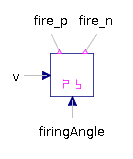Analog Winding - MapleSim Help

Quasi Static Analog Winding

Quasistatic single phase winding neglecting induced voltageDescription The Quasi Static Analog Winding component consists of a single-phase electromagnetic coupling with a resistor in series with the electric winding.Connections

 Name Description Modelica ID ${\mathrm{pin}}_{p}$ Positive electrical pin pin_p ${\mathrm{pin}}_{n}$ Negative electrical pin pin_n ${\mathrm{port}}_{n}$ Negative complex magnetic port port_n ${\mathrm{port}}_{p}$ Positive complex magnetic port port_p $\mathrm{heatPortWinding}$ Heat ports of winding resistor heatPortWindingParameters

 Name Default Units Description Modelica ID $\mathrm{\alpha }$ $0$ $\frac{1}{K}$ Temperature coefficient of winding at 20 degC alpha20 ${R}_{\mathrm{ref}}$ $\mathrm{\Omega }$ Winding resistance per phase at ${T}_{\mathrm{ref}}$ RRef ${T}_{\mathrm{ref}}$ $293.15$ $K$ Reference temperature of winding TRef ${T}_{\mathrm{oper}}$ $293.15$ $K$ Operational temperature of winding TOperational ${n}_{\mathrm{eff}}$ $1$ Effective number of turns per phase effectiveTurns Use Heat Port $\mathrm{false}$ True (checked) means heat port is enabled useHeatPortModelica Standard Library The component described in this topic is from the Modelica Standard Library. To view the original documentation, which includes author and copyright information, click here.